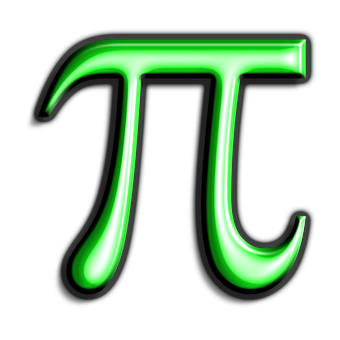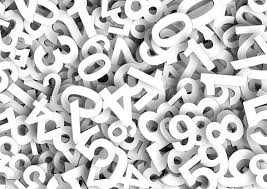HomeAILET UGLearn To Solve Ratio And Proportion for CLAT

# Learn To Solve Ratio And Proportion for CLAT

In any kind of competitive exam, the questions involving ratio and proportion are pretty common. It is also very evident from the Sample papers released by CLAT Consortium that the concept of ratio is one of the important topics that​​ one must cover in order to fetch marks.​​ If you have gone through the CLAT Consortium’s Sample Paper, you must be aware with the changed pattern of questions in the Quantitative Section. All the questions are in the passage form and multiple questions are asked on the basis of information provided through the various special charts like pie chart, bar graph, line chart, tables etc

It is one of the easiest topic in the Quants section and does not involve very complex formula or concepts. If two quatities are​​ of the same type, then only they can be expressed as ratios. Ratio is the number ogf times one quantity contains the another. But the type must be same. You cannot find the ratio between 1 kg of potato and 2 laptops. Ratios are expressed in the form of A:B​​ or A/B ​​ where A is called antecedent and B is called consequent. When two ratios are equal in value, then they are said to be in proportion. The equivalence of two ratios is called proportion. If a : b = c : d, then a, b, c, d are said to be in proportion. Here, a x d = b​​ x c.​​ There are a few points with respect to the ratio and proportion that are to be kept in mind while solving the questions of ratio and proportion. Pay attention to these point and keep them in mind while solving the questions relating​​ to ratio and proportion.

• If p : q = r : s, then p, q, r, s are said to be in proportion. Here, p x s = q x r.

• ​​ If we have two ratios, say p : q and r : s, then (p x r) : (q x s) is called the compounded ratio.

• If p : q = r : s, i.e., p/q = r/s, then (p +​​ q) / (p – q) = (r + s) / (r – s)
This is called Componendo and Dividendo.

• If we say that ‘p’ is directly proportional to ‘q’, it means that p = k x q, where ‘k’ is the constant of proportionality.

• If we say that ‘p’ is inversely proportional to ‘q’, it implies that p = k / q or p x q = k, where ‘k’ is the constant of proportionality

• If a ratio is multiplied or divided by a certain number, the properties of the ratio do not change. For example, if we multiply 2 : 3 by 7, we get 14 : 21, which is same as 2:3.

As hyou must be aware that Hardwork and smartwork go hand in hand. Therefore we have to focus our preparation as per the changed pattern in the CLAT Sample paper released by CLAT Consortium pon their website. Therefore, there are passage based questions which involve the concept of Percentage, Average, Ratio, Simple Interest, Compound Interest, Profit & Loss etc.So, let us focus on the examples given below in order to make sure that we are quick at solving the questions that involve the concept of Ratio and proportion:

Example 1:​​ The ratio of Rice and Pulses in an Indian one pot dish ‘Khichdi’ is 13 : 7. How much rice will be there in 100 kg of Khichdi?

​​ ​​ ​​ ​​ ​​​​ Solution: ​​ This is the simplest and the most diresct kind of question. To find​​ How much  ​​ ​​​​ rice will be there in 100 kg of Khichdi,

​​ Since, 13+7 = 20

 Therefore, ​​ Amount of rice = ( 13/20) *100 ​​ = 65 kg​​

Example 2: A mixture contains artificial blood and orange juice in the ratio 4: 3. If 10 litres of orange juice is added to the mixture, the​​ ratio becomes 4: 5. Find the initial quantity of artificial blood in the given mixture.

Solution: The initial ratio is 4 : 3.
Let ‘k’ be the common ratio.
=> Initial quantity of artificial blood ​​ = 4 k
=> Initial quantity of orange juice = 3 k
=>​​ Final quantity of artificial blood ​​ = 4 k
=> Final quantity of orange juice = 3 k + 10
Final ratio = 4 k : 3 k + 10 = 4 : 5
=> k = 5
Therefore, initial quantity of artificial blood ​​ in the given mixture = 4 k = 20 litersExample 3: Three friends Abhilasha​​ , Asad and Aditi ​​ started a business, each investing Rs. 10,000. After 5 months Abhilasha withdrew Rs. 3000, Asad ​​ withdrew Rs. 2000 and Aditi invested Rs. 3000 more. At the end of the year, there was a total profit of Rs. 34,600. Find the share of Abhilasha , Asad and Aditi ​​ separately.

Solution :​​ We know that if the period of investment is not uniform, the gains/losses from the business are divided in the ratio of their inputs, where input is calculated as the product of amount of investment and time period of investment.
So, input = value of investment x period of investment, and here, period of investment would be broken into parts as the investment is not uniform throughout the time period.
Abhilasha’s input = (10,000 x 5) + (7,000 x 7) = 99,000
Asad’s​​ input = (10,000 x 5) + (8,000 x 7) = 1,06,000
Aditi’s input = (10,000 x 5) + (13,000 x 7) = 1,41,000
=> Abhilasha : Asad : Aditi = 99000 : 106000 : 141000
=> Abhilasha : Asad : Aditi = 99 : 106 : 141
=> Abhilasha : Asad : Aditi = (99 / 346) : (106 / 346)​​ : (141 / 346)
Thus, Abhilasha’s share = (99 / 346) x 34600 = Rs. 9900
Asad’s share = (106 / 346) x 34600 = Rs. 10600
Aditi’s share = (141 / 346) x 34600 = Rs. 14100

Example 4:​​ In a certain government department, the ratio of male employees to female employees is 7: 5. If there are 2400 employees in the department, then how many female employees are there?

Solution:​​ Let the number of male employees and female employees are 7x and 5x, respectively.
Given, total number of employees = 2400
​​ 7x + 5x = 2400 ​​   12x = 2400
x = 200
​​     number of female employees = 5x  =  5 × 200 = 1000

Example 5: 5 bottles as much as 12 tiffin, 7 tiffin as much as 2 box, 3 box as much as 2 bags. If the cost of 5 bags is Rs 875, then find the cost of a bottle.

Solution:​​ 5​​ bags = 875
2 bags = 875 * 2/5 = 3 box
2 box cost= 875 * 2/5 * 2/3 = 7 tiffin cost
12 tiffin cost = 875 * 2/5 * 2/3 * 12/7 = 5 bottles
1 bottle = 875 * 2/5 * 2/3 * 12/7 * 1/5 =​​ Rs 80

Example 7:​​ The ratio of the age of Mayank and his Priyanshi is 6:5. After​​ 16 years, the ratio becomes 10:9. Find Mayank’s age when the Priyanshi was born.

Solution:​​ Let x is Mayank’s age
And y is the Priyanshi’s age.
x/y= 6/5
x=(6/5)y

After 16 years,
(x + 16)/(y + 16) = 10/9
9x + 144 = 10y + 160
9*(6/5)y = `10y + 16
54y – 50y =​​ 80
y=20
Then x = 24
When Priyanshi was born Mayank’s age =​​ 4 years.